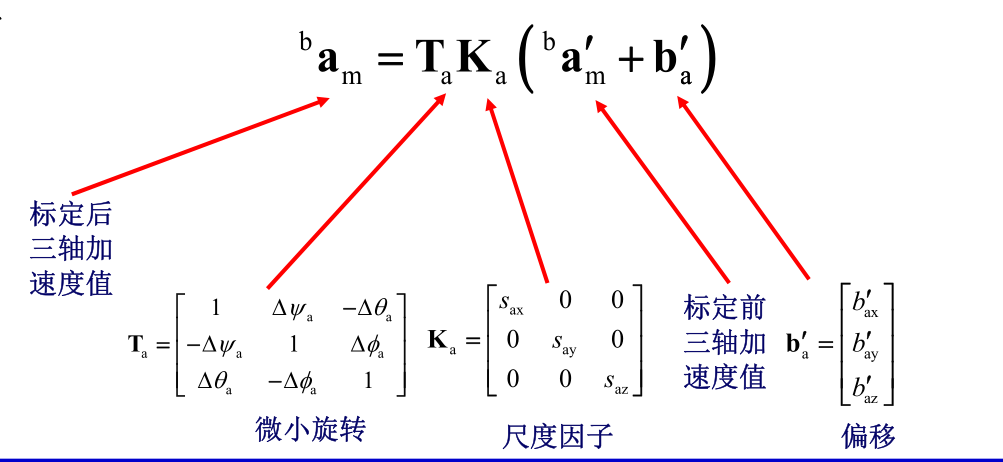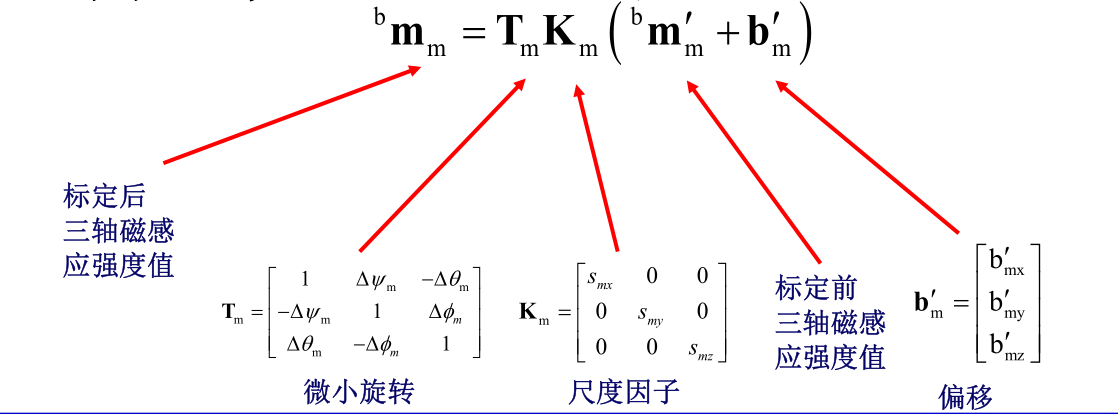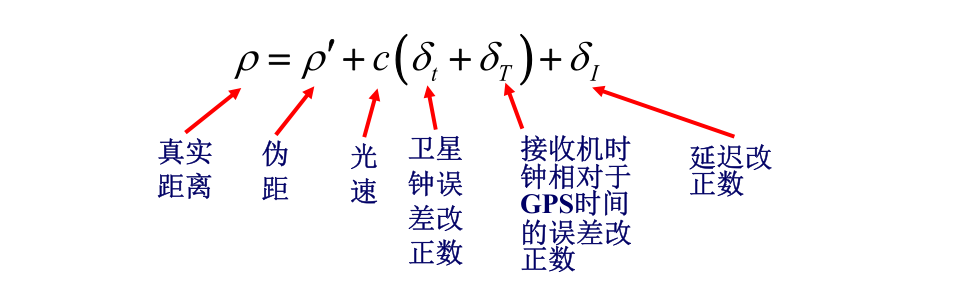V1

2022/11/28阅读：72主题：默认主题

# 传感器建模及校准含matlab代码

## 1、加速度计常用标定手段： 1、利用静止状态的加速度计向量的模值始终等于g，可以采取处于静止状态下各个方向的加速度计矢量，利用最小二乘获取上述图片中的9个变量。

2、将加速度各个轴分别向上、向下采集其数据，然后求解上述的模型参数。

• Reference vectors
• accel_corr_ref = [ g 0 0 ] // nose up
•                    | -g  0  0 |     // nose down

•                    |  0  g  0 |     // left side down

•                    |  0 -g  0 |     // right side down

•                    |  0  0  g |     // on back

•                    [  0  0 -g ]     // level

• accel_raw_ref
• accel_corr_ref[i] = accel_T * (accel_raw_ref[i] - accel_offs), i = 0...5
• 6 reference vectors * 3 axes = 18 equations
• 9 (accel_T) + 3 (accel_offs) = 12 unknown constants
• Find accel_offs
• accel_offs[i] = (accel_raw_ref[i2][i] + accel_raw_ref[i2+1][i]) / 2
• Find accel_T
• 9 unknown constants
• need 9 equations -> use 3 of 6 measurements -> 3 * 3 = 9 equations
• accel_corr_ref[i2] = accel_T * (accel_raw_ref[i2] - accel_offs), i = 0...2
• Solve separate system for each row of accel_T:
• accel_corr_ref[j2][i] = accel_T[i] * (accel_raw_ref[j2] - accel_offs), j = 0...2
• A * x = b
• x = [ accel_T[i] ]
• | accel_T[i] |

• [ accel_T[i] ]

• b = [ accel_corr_ref[i] ] // One measurement per side is enough
• | accel_corr_ref[i] |

• [ accel_corr_ref[i] ]

• a[i][j] = accel_raw_ref[i][j] - accel_offs[j], i = 0;2;4, j = 0...2
• Matrix A is common for all three systems:
• A = [ a a a ]
• | a  a  a |

• [ a  a  a ]

• x = A^-1 * b
• accel_T = A^-1 * g

## 3、磁力计根据采集的充足磁力计数据，将其拟合成球形或者椭球形。然后带入上述公式获取其对应系数。

## 6、GPS做紧耦合定位时可以利用上述公式作为测量方程。

## 关注公众号，获取惯导解算代码、传感器模型代码以及imu的allan方差分析代码

❤️ 扫一扫，添加我的公众号或者搜索【无人机开发】V1